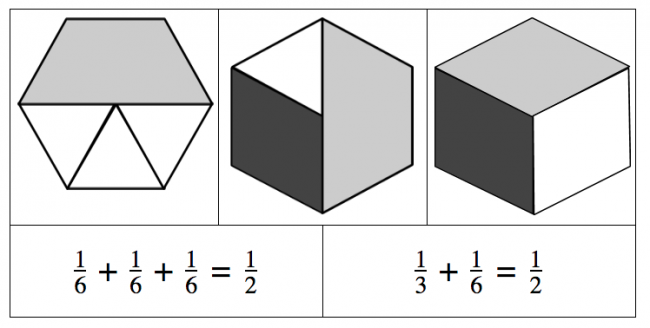## You are here

Preview:This task focuses on the relationship between the size of friendly fractions like 1/2, 1/3, and 1/6. In particular students should see that these fractions are in ratios of each other and that 1/6 is the smallest fraction with 1/2 being the largest.

Connecting Representations
Big Idea:
Grade 8: Number represents, describes, and compares the quantities of ratios, rates, and percents.
Grade 8: Computational fluency and flexibility extend to operations with fractions.
Content:
using pattern blocks or Cuisenaire Rods adding fractions relative size of fractions
Curricular Competency:
Reasoning and analyzing: Develop mental math strategies and abilities to make sense of quantities
Understanding and solving: Develop, demonstrate, and apply mathematical understanding through play, inquiry, and problem solving
Understanding and solving: Visualize to explore mathematical concepts
Understanding and solving: Develop and use multiple strategies to engage in problem solving
Communicating and representing: Communicate mathematical thinking in many ways
Communicating and representing: Use mathematical vocabulary and language to contribute to mathematical discussions
Communicating and representing: Explain and justify mathematical ideas and decisions
Communicating and representing: Represent mathematical ideas in concrete, pictorial, and symbolic forms
Connecting and reflecting: Reflect on mathematical thinking

### Fractions

For younger children, in order for them to familiarize with fraction, they have to immerse on activities related to fraction. That's why most of the activities are games that are related to fractions. But the most effective activity that I have ever experienced is something related with food. For example, eating pizza will make them familiar with fraction. You have to divide pizza among them and teach them the parts of pizza in relation with fraction.

For higher grades students, familiarization with the rules of addition, subtraction, multiplication, and division of fraction is the most important. To familiarize the rules, they have to practice it diligently. It takes time to practice fraction and mastering them would require serious effort.

To get the best result in short time while practicing fraction, they need to have a checker that would confirm if their answer is correct or not. The best checker that I know is the fraction calculator from fractioncalc.com. I understand that some would prefer to do the checking manually by repeating the equation several times. This is also good but takes time. If one could make correct calculation at once by confirming the answer through calculator, it would be much better and can practice several equation in short time.